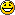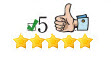# Little Acorns Review Updated For June 2019Only Our 2nd EVER 5 Stars and Highly Recommended Product!Little Acorns
is a low-liability laying formula that uses low stakes to grow bigger banks and be in the position of being confident to increase your stakes as the bank increases.

The system has been developed by Andrew David, who is confident that your betting balance will increase between seven and 35 times each month, without exposing yourself to high liabilities.

It was a pretty major decision to do this but over 6 years we decided that Little Acorns was worthy of only our 2nd 5 Star Rating on BST. It is very hard to achieve this ranking and i often wondered over the years whether it would be a backing system or a laying system that achieved 5 star status next to All By The Book. Obviously since Little Acorns was given this accolade Zero Risk Trader has also achieved 5 star status and sits with Little Acorns and All By The Book. The difference however is that All By The Book & Zero Risk Trader are systems that are guaranteed to work all year round as it relies on flaws in the betting world and is not dependant on the user. Where as Little Acorns relies on its method staying profitable and is straight bets. This means that in 6 years Little Acorns is (as of April 2014) the only straight betting product that is highly recommended.

I have watched and admired this service since it was released and year on year it makes a profit which is very difficult to do.

For an out and out laying service i haven’t seen one better in all my time on line reviewing systems and services.

If you did not know, Little Acorns is a low-liability laying formula that uses low stakes to grow bigger banks and be in the position of being confident to increase your stakes as the bank increases.

The system has been developed by Andrew David, who is confident that your betting balance will increase between seven and 35 times each month, without exposing yourself to high liabilities.

Andrew has said that anyone who purchases through the link below will get access to his Bonus Place Lay system (which is a fantastic bonus) if you ask him for it after purchase. Alex who left a testimonial below the results describes it as phenomenal!

The Live Review Results Recorded On This Blog 🙂
Opens in new window Page 29 read from bottom to top…

### Our Live Review Results;

Wherever possible we try to monitor our 5* products indefinitely. These products will be updated as often as we can manage. We have had a custom built bot made which we take the figures from and verify them with the product vendor. This is so that we can be sure Little Acorns deserves to remain a 5 Star product for our readers.

### The Results since the start of our review in October 2012 are as follows;

MAY 17TH 2012 TO OCTOBER 31ST 2012 =  +34.19 (includes 2 small losing months)
NOVEMBER: 2012 = +8.20
DECEMBER: 2012 = +7.70
JANUARY: 2013 = +9.80
FEBRUARY: 2013 = +7.15
MARCH:  2013 = +11.10
APRIL: 2013 = +12.55
MAY: 2013 = -5.90
JUNE: 2013 = +15.20
JULY: 2013 = +17.40
AUGUST: 2013 =  +16.70
SEPTEMBER: 2013 = +19.50
OCTOBER: 2013 = +10.80
NOVEMBER: 2013 = +3.33
DECEMBER: 2013 = +11.11
JANUARY: 2014 = +6.68
FEBRUARY: 2014 = +14.65
MARCH: 2014 = +11.10
APRIL: 2014 = +2.33
MAY: 2014 = +7.20
JUNE: 2014 = +11.77
JULY: 2014 = +13.30
AUGUST: 2014 = +7.73
SEPTEMBER: 2014 = +8.85
OCTOBER: 2014 = +8.08
NOVEMBER: 2014 = +10.90
DECEMBER: 2014 = +15.20
JANUARY: 2015 = +11.25
FEBRUARY: 2015 = +13.25
MARCH: 2015 = +13.22
APRIL: 2015 = +2.00
MAY: 2015 = +13.10
JUNE: 2015 = -3.79
JULY: 2015 = +9.20
AUGUST: 2015 = +9.90
SEPTEMBER: 2015 = +14.10
OCTOBER: 2015 = +8.87
NOVEMBER: 2015 = +12.60
DECEMBER: 2015 = +7.90
JANUARY: 2016 = +11.90
FEBRUARY: 2016 = +9.30
MARCH: 2016 = +8.85
APRIL: 2016 = +10.70
MAY: 2016 = +12.60
JUNE: 2016 = -2.65
JULY: 2016 = -7.65

AUGUST: 2016 = +8.80
SEPTEMBER: 2016 = +13.30
OCTOBER: 2016 = +11.20
NOVEMBER: 2016 = +10.40
DECEMBER: 2016 = +8.95
JANUARY: 2017 = +10.10
FEBRUARY: 2017 = +12.05
MARCH: 2017 = +11.55
APRIL: 2017 = +11.40
MAY: 2017 = +11.00
JUNE: 2017 = +9.90
JULY: 2017 = -7.90
AUGUST: 2017 = +11.05
SEPTEMBER: 2017 = +14.10
OCTOBER: 2017 = +7.95
NOVEMBER: 2017 = +13.15
DECEMBER: 2017 = -7.75
JANUARY: 2018 = +12.15
FEBRUARY: 2018 = +9.50
MARCH: 2018 = +8.45
APRIL: 2018 = +7.05
MAY: 2018 = +10.15
JUNE: 2018 = +11.85
JULY: 2018 = -11.50
AUGUST: 2018 = +6.95
SEPTEMBER: 2018 = +14.15
OCTOBER: 2018 = +12.40
NOVEMBER: 2018 = +13.30
DECEMBER: 2018 = +10.90
JANUARY: 2019 = +3.40
FEBRUARY: 2019 = +5.70
MARCH: 2019 = -4.10
APRIL: 2019 = +11.05
MAY: 2019 = -13.11
JUNE: 2019 = +7.95

85 Months Total Is +715.85 Points Profit! [FULLY PROOFED! :-D]
Only 11 losing months in 85 months…

### The Results Using Two Steps Back Staking Plan

We have also been following the system using an alternative staking plan called Two Steps Back that is now included with the system. We’ve been following it since February and it has been outperforming the original staking plan. We are no longer using Two Steps Back although it continues to perform well.

FEBRUARY: 2014 = +18.11
MARCH: 2014 = +15.99

APRIL: 2014 = +12.35
MAY: 2014 = +13.10
JUNE: 2014 = +15.90
JULY: 2014 = +16.45
AUGUST: 2014 = +11.12
SEPTEMBER: 2014 = -1.57
OCTOBER: 2014 = +13.33
NOVEMBER: 2014 = +13.90
DECEMBER: 2014 = +18.24
JANUARY: 2015 = +16.00
FEBRUARY: 2015 = +15.75
MARCH: 2015 = +15.72
APRIL: 2015 = +2.75
MAY: 2015 = +16.60
JUNE: 2015 = +0.00
JULY: 2015 = +10.56
AUGUST: 2015 = +12.00
SEPTEMBER: 2015 = +17.61
OCTOBER: 2015 = +11.11
NOVEMBER: 2015 = +15.15
DECEMBER: 2015 = +15.15
JANUARY: 2016 = +12.70
FEBRUARY: 2016 = +10.95
MARCH: 2016 = +10.15
APRIL: 2016 = +11.40
MAY: 2016 = +15.20

28 Months Total Is +352.52 Points Profit (compared to +275.15 Points Profit in same period using original staking plan)
Only 1 losing month in 28 months…

### Bonus Place Lay Results

Some buyers were also able to claim a bonus system which is a Place Lay System. So here are the last results.

MARCH: 2014 = +18.23
APRIL: 2014 = +22.15
MAY: 2014 = +42.05
JUNE: 2014 = +7.95
JULY: 2014 = +14.40
AUGUST: 2014 = +29.30
SEPTEMBER: 2014 = +12.10
OCTOBER: 2014 = +19.90
NOVEMBER: 2014 = +11.90
DECEMBER: 2014 = +16.30
JANUARY: 2015 = -6.60
FEBRUARY: 2015 = +18.70
MARCH: 2015 = +7.90
APRIL: 2015 = +5.40
MAY: 2015 = +18.55
JUNE: 2015 = +11.10
JULY: 2015 = +16.25
AUGUST: 2015 = +15.20
SEPTEMBER: 2015 = +15.60
OCTOBER: 2015 = +9.31
NOVEMBER: 2015 = +11.90
DECEMBER: 2015 = +17.05
JANUARY: 2016 = -13.70
FEBRUARY: 2016 = +12.30
MARCH: 2016 = +5.50
APRIL: 2016 = +7.90
MAY: 2016 = +3.40
JUNE: 2016 = +2.25
JULY: 2016 = +13.05
AUGUST: 2016 = +5.50
SEPTEMBER: 2016 = +8.75
OCTOBER: 2016 = +14.05
NOVEMBER: 2016 = +9.80
DECEMBER: 2016
= +12.25
JANUARY: 2017 = +7.15
FEBRUARY: 2017 = +14.40
MARCH: 2017 = +12.75
APRIL: 2017 = +11.90
MAY: 2017 = +10.10
JUNE: 2017 = -7.10

JULY: 2017 = +11.90
AUGUST: 2017 = +10.60
SEPTEMBER: 2017 = +17.61
OCTOBER: 2017 = +11.80
NOVEMBER: 2017 = +15.05
DECEMBER: 2017
= +7.15
JANUARY: 2018 = +7.05
FEBRUARY: 2018 = +11.25
MARCH: 2018 = +4.50
APRIL: 2018 = +6.85
MAY: 2018 = +11.10
JUNE: 2018 = +17.65
JULY: 2018 = -7.05
AUGUST: 2018 = +16.95
SEPTEMBER: 2018 = +9.90
OCTOBER: 2018 = +2.10
NOVEMBER: 2018
= +8.95
DECEMBER: 2018 = +13.05
JANUARY: 2019 = +6.60
FEBRUARY: 2019 = +8.80
MARCH: 2019 = +4.30
APRIL: 2019 = +14.90
MAY: 2019 = +11.15
JUNE: 2019 = +9.45

64 Months Total Is +696.50 Points Profit
Only 4 losing months in 64 months…

### Testimonial from some of our most recent readers;

Just a quick update. Don’t get me wrong, the original little acorns strategy is fine and proving a nice slow burner to date. But the place laying strategy currently seems to be absolutely phenomenal.

Really looking forward to seeing how this pans out in the longer run because January 2014 and December 2013 have both been very profitable.

PS Still going strong on Football Bankbuilder. With discipline I don’t see how these systems can fail in the long run (but I suppose you already know that). Really suits my preferred approach as well.

Cheers,Alex

============

I have been very fortunate to join Little Acorns at a highly profitable time. Since starting on September 9th I’ve had 19 winning bets and 9 losing bets. I understand that you are willing to supply a few additional “tweaks” and pieces of advice about optimum use of the system and would be grateful to receive them. Also, I would welcome any advice about using a bot for Little Acorns.

Regards
David M